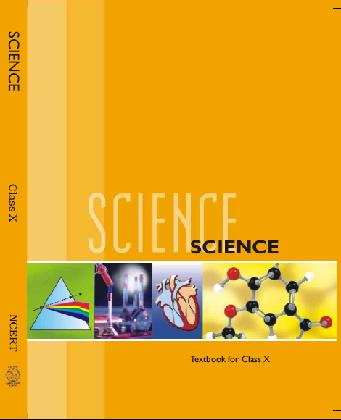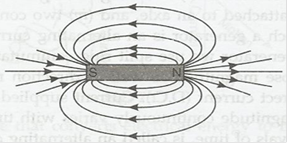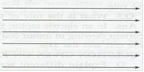# NCERT Solutions for Class 10 Science Magnetic Effects of Electric Current Part 1## myCBSEguide App

CBSE, NCERT, JEE Main, NEET-UG, NDA, Exam Papers, Question Bank, NCERT Solutions, Exemplars, Revision Notes, Free Videos, MCQ Tests & more.

NCERT solutions for Class 10 Science Magnetic Effects of Electric Current## NCERT Class 10 Science Chapter wise Solutions

• 1 – Chemical Reactions and Equations
• 2 – Acids, Bases and Salts
• 3 – Metals and Non-metals
• 4 – Carbon and Its Compounds
• 5 – Periodic Classification of Elements
• 6 – Life Processes
• 7 – Control and Coordination
• 8 – How do Organisms Reproduce?
• 9 – Heredity and Evolution
• 10 – Light Reflection and Refraction
• 11 – Human Eye and Colourful World
• 12 – Electricity
• 13 – Magnetic Effects of Electric Current
• 14 – Sources of Energy
• 15 – Our Environment
• 16 – Management of Natural Resources

## NCERT Solutions for Class 10 Science Magnetic Effects of Electric Current Part 1

###### 1. Why does a compass needle get deflected when brought near a bar magnet?

Ans. The compass needle is small bar magnet. When a compass needle is brought near a bar magnet then due to repulsive force between unlike poles and attraction between unlike poles, the compass needle is deflected and settle in the direction of net magnetic field.

NCERT Solutions for Class 10 Science Magnetic Effects of Electric Current Part 1

###### 1. Draw magnetic field around a bar magnet.

Ans. Magnetic field lines are as follows:###### 2. List the properties of magnetic lines of force.

Ans. Properties of magnetic field lines of force as follows:

a. Outside a magnet, the field lines are directed from N-pole of magnet towards S-pole and inside the magnet lines are directed form S-pole to N-pole.

b. Magnetic field lines are closed curves.

c. No two magnetic field lines intersect each other’s.

d. Relative strength of magnetic field lines is given by degree of closeness of the filed lines.

###### 3. Why don’t two magnetic lines of force intersect each other?

Ans. No, two magnetic field lines can ever intersect each other. If they do, then it would mean that at the point of intersection there are two directions of magnetic field, which is not possible.

NCERT Solutions for Class 10 Science Magnetic Effects of Electric Current Part 1

###### 1. Consider a circular loop of wire lying in the plane of the table. Let the current pass through the loop clockwise. Apply the right-hand rule to find out the direction of the magnetic field inside and outside the loop.

Ans. As per right-hand rule, we find that inside the loop, the magnetic field lines are directed perpendicular to the plane of paper in the inward direction. Outside the loop magnetic field lines are directed out of the plane paper.

NCERT Solutions for Class 10 Science Magnetic Effects of Electric Current Part 1

###### 2. The magnetic field in a given region is uniform. Draw a diagram to represent it.

Ans. The uniform magnetic field is represented by parallel equispaced lines of equal length as follows:NCERT Solutions for Class 10 Science Magnetic Effects of Electric Current Part 1

###### 3. Choose the correct option:

The magnetic field inside a long straight solenoid-carrying current

(a) Is zero.

(b) Decrease as we move towards its end.

(c) Increase as we move towards it end.

(d) Is the same all points.

Ans. (d) is the same at all points.

NCERT Solutions for Class 10 Science Magnetic Effects of Electric Current Part 1
1. Which of the following property of a proton can change while it moves freely in a magnetic field?

(a) mass

(b) speed

(c) velocity

(d) momentum

Ans. (c), (d) Velocity as well as momentum will change.

NCERT Solutions for Class 10 Science Magnetic Effects of Electric Current Part 1

###### 2. In activity 13.7, how do we think the displacement of rod AB will be affected if (i) current is rod AB is increased, (ii) a stronger horse shoe magnet is used, and (iii) length of the rod AB is increased?

Ans. (i) O increasing the current in rod AB its displacement will increase.

(ii) If stronger horse-shoe magnet is used then the displacement of rod AB will increase.

(iii) If length of the rod is increased, force acting on it will increase and hence, displacement of the rod increases.

NCERT Solutions for Class 10 Science Magnetic Effects of Electric Current Part 1

###### 3. A positively-charged particle (alpha-particle) projected towards west is deflected towards north by a magnetic field. The direction of magnetic field is :

(a) towards south

(b)towards east

(c) downward

(d) upward

Ans. (d) the direction of magnetic field is vertically upward.

NCERT Solutions for Class 10 Science Magnetic Effects of Electric Current Part 1

###### 1. State Fleming’s left-hand rule.

Ans. Fleming’s left hand rule states that stretch the forefinger, the central finger and the thumb of your left hand mutually perpendicular to each other. If the forefinger shows the direction of the magnetic field and central finger that of the current, then the thumb will point towards the direction of motion of the conductor.

NCERT Solutions for Class 10 Science Magnetic Effects of Electric Current Part 1

###### 2. What is the principle of an electric motor?

Ans. An electric motor is based on the principle that the current carrying conductor experiences a force when placed in a magnetic field. If the direction of the magnetic field and that of the current are mutually perpendicular then the direction of the force is given by Fleming’s left-hand rule.

###### 3. What is the role of the split ring in an electric motor?

Ans. In electric motor, the split ring acts as commutator. Due to its action, the direction of current flowing in motor coil reverses after half turn, giving rise to a continuous rotation of the coil and the axle.

NCERT Solutions for Class 10 Science Magnetic Effects of Electric Current Part 1

###### 1. Explain different ways to induce current in a coil.

Ans. Different ways to induce current in a coil are as follows:

(a) If a magnetic field is changed around a coil then an induced current is set up in the coil.

(b) If a coil is moved in magnetic field, then again an induced current is set up in the coil.

(c) If a coil is rotated in a uniform magnetic field.

NCERT Solutions for Class 10 Science Magnetic Effects of Electric Current Part 1

###### 1. State the principle of an electric generator.

Ans. An electric generator is based on the principle of electromagnetic induction. When a rectangular coil is rotated in a uniform magnetic field, an induced emf is generated between the ends of the coil.

###### 2. Name some sources of direct current.

Ans. Some sources of direct current are a cell, a battery and a D.C. generator.

###### 3. Which sources produce alternating current?

Ans. A.C. generator and common inverter used in houses for emergency power supply produce alternating current.

NCERT Solutions for Class 10 Science Magnetic Effects of Electric Current Part 1

###### 4. Choose the correct option:

A rectangular coil of copper wires is rotated in a magnetic field. The direction of the induced current changes once in each

(a) two revolutions

(b) one revolution

(c) half revolution

(d) one-fourth revolution

Ans. (c) half-rotation.

NCERT Solutions for Class 10 Science Magnetic Effects of Electric Current Part 1

###### 1. Name two safety measures commonly used in electric circuits and appliances.

Ans. Two safety measures are:

(a) use of earth wire and proper earthing.

(b) use of fuse.

NCERT Solutions for Class 10 Science Magnetic Effects of Electric Current Part 1

###### 2. An electric oven of 2 kW power rating is operated in a domestic electric circuit (220V) that has a current rating of 5.A. What result do you expect? Explain.

Ans. Power rating of electric oven (P) = 2 kW = 2000 W

Current drawn (I) = P/V = 2000/220 = 9.09 A.

As the current rating of domestic electric circuit is only 5A the oven draws a current of 9.09 A. Which is more than the current rating; hence the circuit will be damaged due to overheating/overloading.

NCERT Solutions for Class 10 Science Magnetic Effects of Electric Current Part 1

###### 3. What precaution should be taken to avoid the overloading of domestic electric circuit?

Ans. We should take following precaution to avoid the overloading of domestic electric circuit:

(a) Two separate circuits should be used, one of 5A current rating of bulbs, fans, tubes etc. and the other 15 A current rating for appliances with higher current rating such as geysers, air coolers, electric iron, electric stoves etc.

(b) Too many appliances should never be connected to a single socket.

(c) A fuse of appropriate current rating should be used with the electric circuit.

## NCERT Solutions for Class 10 Science

NCERT Solutions Class 10 Science PDF (Download) Free from myCBSEguide app and myCBSEguide website. Ncert solution class 10 Science includes text book solutions from Book. NCERT Solutions for CBSE Class 10 Science have total 16 chapters. 10 Science NCERT Solutions in PDF for free Download on our website. Ncert Science class 10 solutions PDF and Science ncert class 10 PDF solutions with latest modifications and as per the latest CBSE syllabus are only available in myCBSEguide.

## CBSE app for Students

To download NCERT Solutions for class 10 Social Science, Computer Science, Home Science,Hindi ,English, Maths Science do check myCBSEguide app or website. myCBSEguide provides sample papers with solution, test papers for chapter-wise practice, NCERT solutions, NCERT Exemplar solutions, quick revision notes for ready reference, CBSE guess papers and CBSE important question papers. Sample Paper all are made available through the best app for CBSE students and myCBSEguide website.### 2 thoughts on “NCERT Solutions for Class 10 Science Magnetic Effects of Electric Current Part 1”

1. Your solution is very good

2. This is helping me a lot I jst have my exam after 2 days